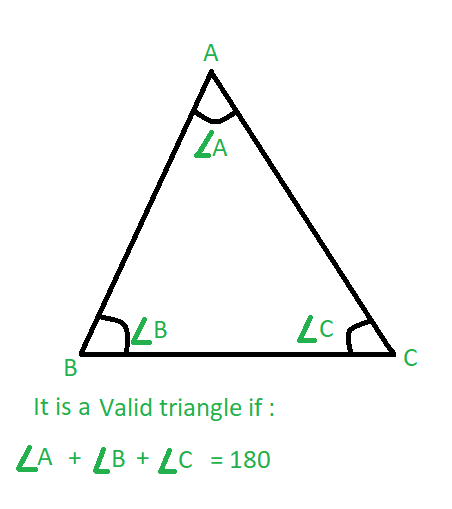# Check whether the triangle is valid or not if angles are given

• Difficulty Level : Basic
• Last Updated : 27 Apr, 2021

Given three integers A, B and C which are the three angles of a possible triangle in degrees, the task is to check whether the triangle is valid or not.
Examples:

Input: A = 60, B = 40, C = 80
Output: Valid
Input: A = 55, B = 45, C = 60
Output: Invalid

Approach: A triangle is valid if the sum of the three angles is equal to 180 degrees.Below is the implementation of the above approach:

## C++

 `// C++ implementation of the approach``#include ``using` `namespace` `std;` `// Function to check if sum of the``// three angles is 180 or not``bool` `Valid(``int` `a, ``int` `b, ``int` `c)``{``    ``// Check condition``    ``if` `(a + b + c == 180 && a != 0 && b != 0 && c != 0)``        ``return` `true``;``    ``else``        ``return` `false``;``}` `// Driver code``int` `main()``{``    ``int` `a = 60, b = 40, c = 80;` `    ``if` `(Valid(a, b, c))``        ``cout << ``"Valid"``;``    ``else``        ``cout << ``"Invalid"``;``}`

## Java

 `// Java program to check``// validity of any triangle``class` `GFG {` `    ``// Function to check if sum of the``    ``// three angles is 180 or not``    ``public` `static` `int` `Valid(``int` `a, ``int` `b, ``int` `c)``    ``{``        ``// check condition``        ``if` `(a + b + c == ``180` `&& a != ``0` `&& b != ``0` `&& c != ``0``)``            ``return` `1``;``        ``else``            ``return` `0``;``    ``}` `    ``// Driver Code``    ``public` `static` `void` `main(String args[])``    ``{` `        ``int` `a = ``60``, b = ``40``, c = ``80``;` `        ``// function calling and print output``        ``if` `((Valid(a, b, c)) == ``1``)``            ``System.out.print(``"Valid"``);``        ``else``            ``System.out.print(``"Invalid"``);``    ``}``}` `// This code is contributed``// by Apurva Sharma`

## Python3

 `# Python3 implementation of the approach` `# Function to check if sum of the``# three angles is 180 or not``def` `Valid(a, b, c):` `    ``# Check condition``    ``if` `((a ``+` `b ``+` `c ``=``=` `180``) ``and` `a !``=` `0` `and` `b !``=` `0` `and` `c !``=` `0``):``        ``return` `True``    ``else``:``        ``return` `False` `# Driver code``if` `__name__ ``=``=` `"__main__"``:``    ``a ``=` `60``    ``b ``=` `40``    ``c ``=` `80``    ``if` `(Valid(a, b, c)):``        ``print``(``"Valid"``)``    ``else``:``        ``print``(``"Invalid"``)` `# This code is contributed by``# sanjeev2552`

## C#

 `// C# program to check``// validity of any triangle``using` `System;` `class` `GFG {` `    ``// Function to check if sum of the``    ``// three angles is 180 or not``    ``public` `static` `int` `Valid(``int` `a, ``int` `b, ``int` `c)``    ``{``        ``// check condition``        ``if` `(a + b + c == 180 && a != 0 && b != 0 && c != 0)``            ``return` `1;``        ``else``            ``return` `0;``    ``}` `    ``// Driver Code``    ``public` `static` `void` `Main()``    ``{``        ``int` `a = 60, b = 40, c = 80;` `        ``// function calling and print output``        ``if` `((Valid(a, b, c)) == 1)``            ``Console.WriteLine(``"Valid"``);``        ``else``            ``Console.WriteLine(``"Invalid"``);``    ``}``}` `// This code is contributed``// by anuj_6`

## Javascript

 `// javascript program to check``// validity of any triangle` `    ``// Function to check if sum of the``    ``// three angles is 180 or not``   ``function` `Valid(a,  b,  c)``    ``{``    ` `        ``// check condition``        ``if` `(a + b + c == 180 && a != 0 && b != 0 && c != 0)``            ``return` `1;``        ``else``            ``return` `0;``    ``}``  ` `    ``// Driver Code``        ``var` `a = 60, b = 40, c = 80;``  ` `        ``// function calling and print output``        ``if` `((Valid(a, b, c)) == 1){``            ``document.write(``"Valid"``);``            ``}``        ``else``{``            ``document.write(``"Invalid"``);``          ``}``    ` `// This code is contributed by bunnyram19.`` `

Output:

`Valid`

My Personal Notes arrow_drop_up